# Living By Chemistry SECOND EDITION Unit 4 TOXINS

• Slides: 14Living By Chemistry SECOND EDITION Unit 4: TOXINS Stoichiometry, Solution Chemistry, and Acids and Bases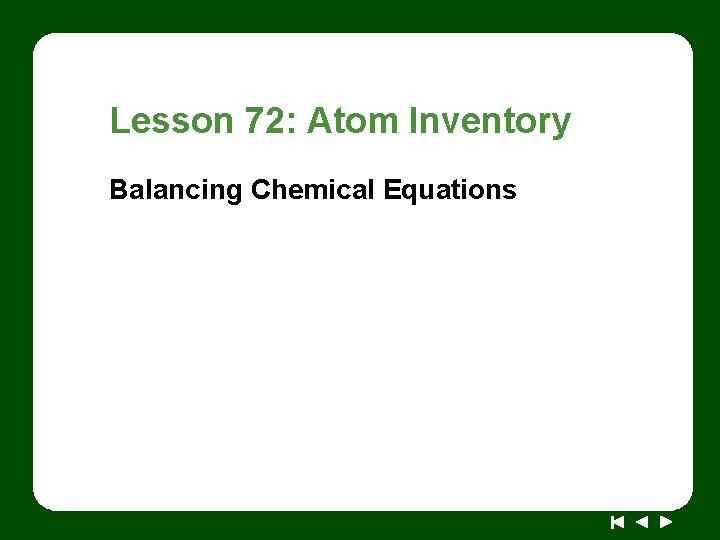Lesson 72: Atom Inventory Balancing Chemical Equations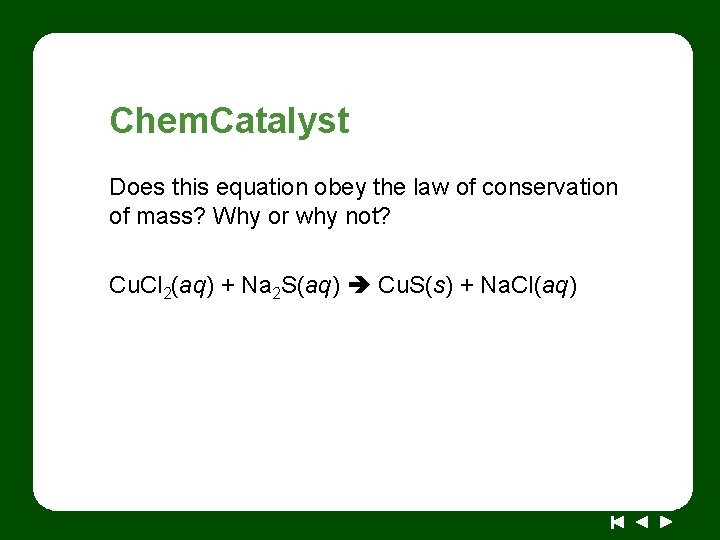Chem. Catalyst Does this equation obey the law of conservation of mass? Why or why not? Cu. Cl 2(aq) + Na 2 S(aq) Cu. S(s) + Na. Cl(aq)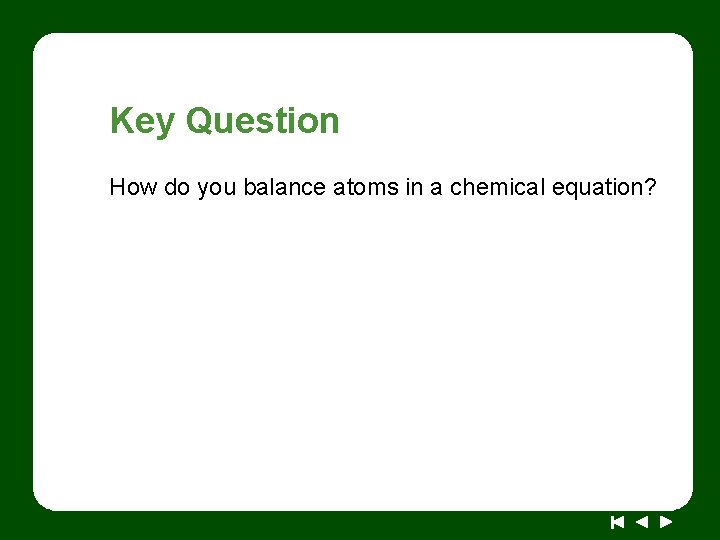Key Question How do you balance atoms in a chemical equation?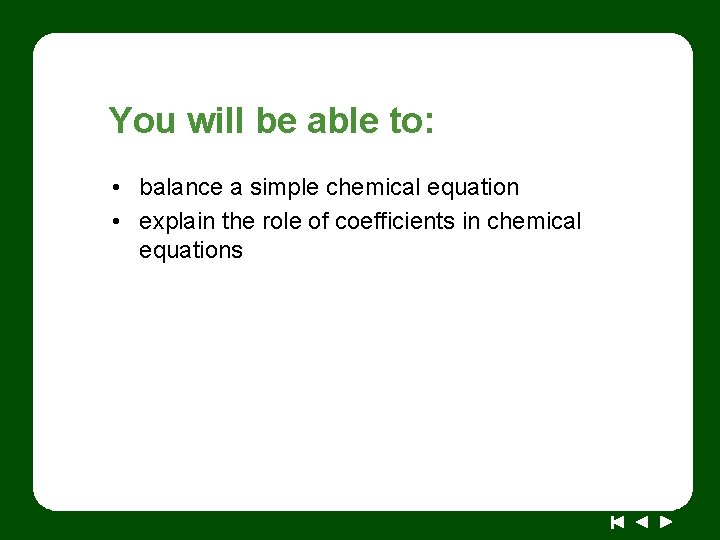You will be able to: • balance a simple chemical equation • explain the role of coefficients in chemical equations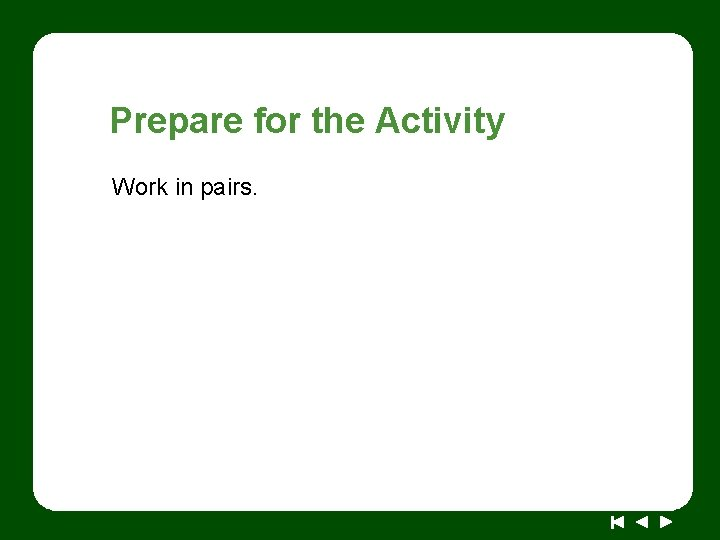Prepare for the Activity Work in pairs.Discussion Notes A balanced chemical equation is one that shows the true mathematical relationship between the reactants and the products in a chemical reaction.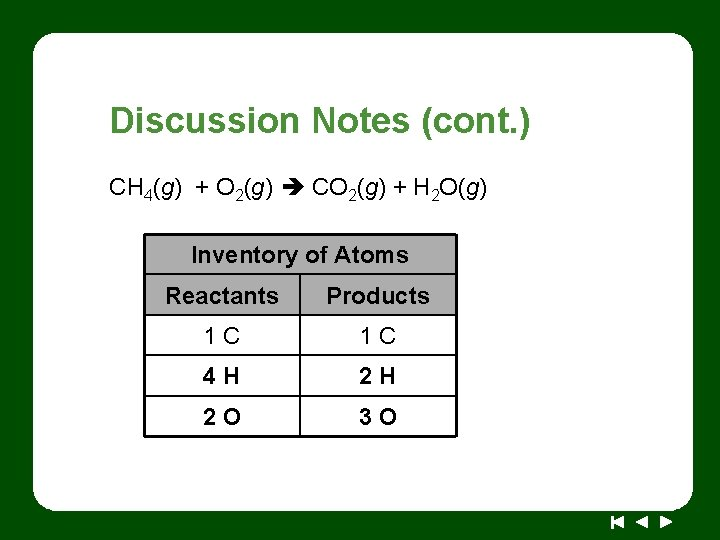Discussion Notes (cont. ) CH 4(g) + O 2(g) CO 2(g) + H 2 O(g) Inventory of Atoms Reactants Products 1 C 1 C 4 H 2 H 2 O 3 ODiscussion Notes (cont. ) CH 4(g) + O 2(g) CO 2(g) + 2 H 2 O(g) Inventory of Atoms Reactants Products 1 C 1 C 4 H 4 H 2 O 4 O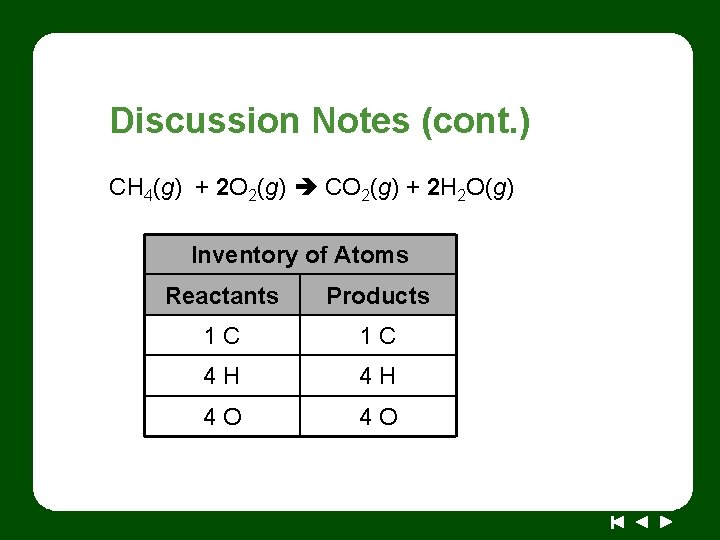Discussion Notes (cont. ) CH 4(g) + 2 O 2(g) CO 2(g) + 2 H 2 O(g) Inventory of Atoms Reactants Products 1 C 1 C 4 H 4 H 4 O 4 ODiscussion Notes (cont. )Discussion Notes (cont. ) There are two types of numbers in a chemical equation: coefficients and subscripts. Coefficients: The coefficients in a chemical equation are the numbers in front of the chemical formulas of the reactants and products. They show the correct ratio in which the reactants combine to form the products. The coefficients in a chemical equation indicate how many “units” of an element or a compound you have.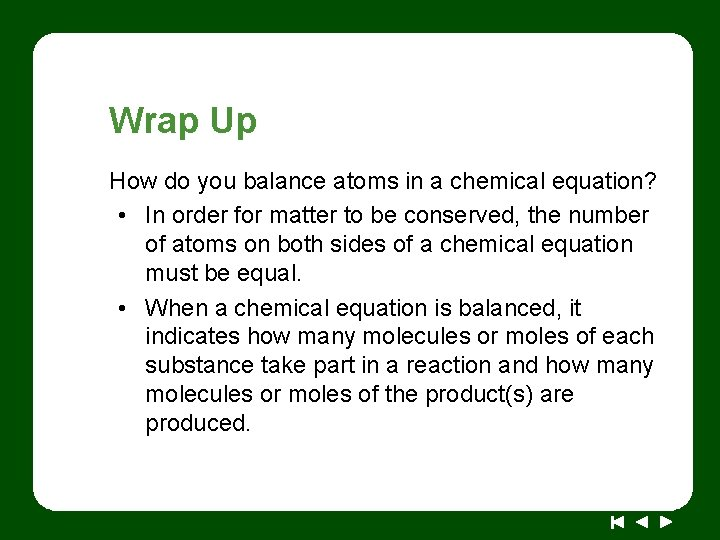Wrap Up How do you balance atoms in a chemical equation? • In order for matter to be conserved, the number of atoms on both sides of a chemical equation must be equal. • When a chemical equation is balanced, it indicates how many molecules or moles of each substance take part in a reaction and how many molecules or moles of the product(s) are produced.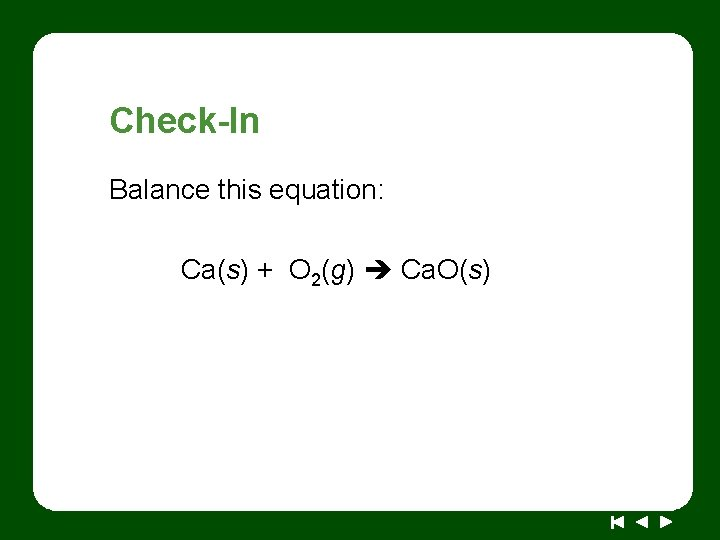Check-In Balance this equation: Ca(s) + O 2(g) Ca. O(s)